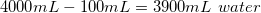# GED Science : Making Solutions

## Example Questions

### Example Question #5 : Chemistry

A student is asked to make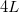of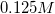NaCl solution. The student is given a stock solution of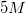NaCl. What volumes of stock NaCl solution and pure water could be used to make the desired final solution?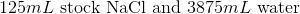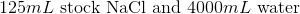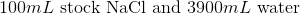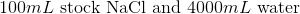Explanation:

Convert liters to mL: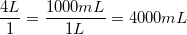This is the TOTAL volume of the solution. Next, determine how much NaCl to add.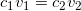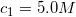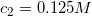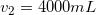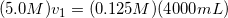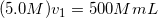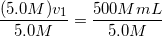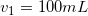Therefore,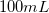of stock NaCl must be added. Subtract this volume from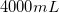to determine the amount of water used in the final solution.